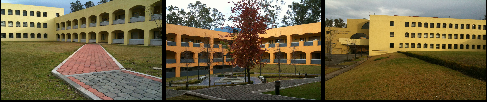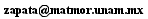José Antonio Zapata
Centro de Ciencias Matemáticas
UNAM Campus Morelia Morelia
CP 58190 Michoacán, MéxicoBefore being here:
Bs Physics UNAM, México
PhD Physics Penn State, USA
Postdoc RRI, India

### Mathematical foundations of canonical loop quantum gravity

When the spacetime is of the form Σ x R, solutions to Einstein’s equation can be constructed from a hamiltonian field theory on Σ, and the group of diffeomorphisms of Σ acts as a gauge-symmetry (it relates points in an extended phase space that are physically indistinguishable). In the framework of loop quantum gravity used in the early 90's, there is an extended space of states in which Σ's diffeomorphism group acts. The construction almost gives rise to an attractive combinatorial structure of knot classes of colored graphs embedded in the Cauchy surface, Σ.

I introduced a specific framework for the kinematics of loop quantum gravity in which gauge-symmetry reduction by automorphisms of Σ leads to a combinatorial structure. In addition, the setting relied on a choice of a PL structure on Σ, and I proved a theorem stating that all PL structures compatible with the smooth structure of Σ lead to the same quantum theory.

A combinatorial approach to diffeomorphism invariant quantum gauge theories
Jose A. Zapata, J.Math.Phys. 38 (1997) 5663-5681
Combinatorial space from loop quantum gravity
Jose A. Zapata, Gen.Rel.Grav. 30 (1998) 1229-1245

Years later, a closely related mathematical setting was used to prove a theorem stating that a type of gauge theory, which includes the Ashtekar-Barbero formulation of general relativity, has a unique "kinematical quantization" which is the one provided by loop quantization. Here is a link to the paper of Lewandowski, Okolow, Sahlmann and Thiemann, and here a link to the paper of Fleischhack. The precise statement of which type of gauge theory is quantized begins by specifying an algebra of kinematical observables; the algebra that is at the basis of loop quantization is referred to as the Holonomy-Flux algebra and was introduced in

Quantum theory of geometry III: Noncommutativity of Riemannian structures
Abhay Ashtekar, Alejandro Corichi, Jose A. Zapata, Class.Quant.Grav. 15 (1998) 2955-2972

The Holonomy-Flux algebra is closely related to that of hamiltonian lattice gauge theory which I knew from my work described in the section "Discrete models for gravity." In this paper the non-commutative nature of the quantum geometry of loop quantum gravity was discovered.